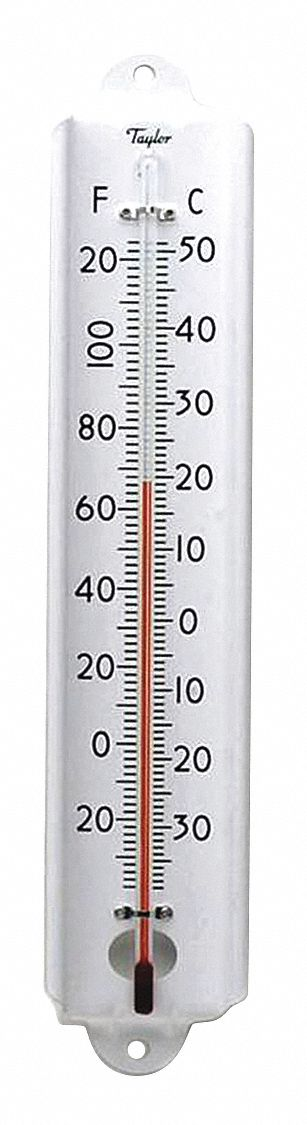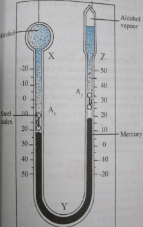# Thermometer

Welcome to class!

In today’s class, we will be talking about the thermometer. Enjoy the class!

### ThermometerA thermometer is an instrument used for measuring temperature. A thermometer usually contains a working substance whose physical properties change with temperature. This is called a thermometric substance. Also, thermometric properties are properties that are sensitive to temperature variation. A thermometer must possess the following qualities:

1. Construction: A thermometer must possess certain properties before it is used. In a mercury thermometer, the thermometer must contain mercury which expands as a result of an increase in temperature.
2. Calibration: A thermometer must be well-calibrated, starting with two reference points. The calibration must be made with shiny ink that can be viewed. For example, in the Celsius scale, the calibration is divided into 100 equal intervals, while the Fahrenheit scale is divided into 180 equal intervals.
3. Sensitivity: A thermometer is said to be sensitive under the following conditions:
• When it can detect even small change in temperature.
• When it shows the temperature of a body in a short time.
• It does not absorb a large amount of heat for its own heating or cooling from the body whose temperature is being measured.

#### Types of thermometer

There are various types of thermometers but the most common ones are:

1. Mercury-in-glass Thermometer
• Clinical Thermometer
• Alcohol-in-glass Thermometer
1. Maximum and Minimum Thermometer
2. Electrical Thermometer
• Platinum resistance thermometer
• Thermocouple thermometer
1. Pyrometers
2. Gas Thermometer
• ##### Mercury-in-glass thermometer:

This is about the commonest type of thermometer. The working substance (thermometric liquid) is mercury. As temperature changes, the volume of the mercury in its tube also changes. This result, in the change of the length of the mercury column in the capillary tube of the thermometer. This is read on the scale on the stem of the thermometer. This type of thermometer can measure the temperature between 39C and 357C. These are the freezing and boiling point of mercury respectively.

###### Clinical thermometer:

A typical example of a mercury-in-glass thermometer is the clinical thermometer. Unlike the ones used in the school laboratory, the clinical thermometer has a short stem. Its temperature range is between 35°C and 43°C. This is because it measures the temperature of a human being. For a healthy human, normal body temperature is about 36.9°C (or 37°C).Clinical thermometer

Apart from the short stem, another feature, which distinguishes clinical thermometer from laboratory thermometer, is the presence of construction at the base of its stem. This prevents the mercury from flowing back into the bulb until when a reading is taken. The mercury is forced back into the bulb by jerking or by shaking the thermometer vigorously.

###### Alcohol-in-glass thermometer:

This is similar to mercury-in-glass thermometer except that its thermometric liquid is alcohol. Alcohol has a much lower freezing point than mercury (-115C). Hence, alcohol-in-glass thermometer is more suitable for low-temperature measurement. However, alcohol boils at 78C and so the alcohol-in-glass thermometer is unsuitable for measuring temperature higher than 78C. This is a serious disadvantage.

Apart from this, mercury has some advantage over alcohol as a thermometric liquid. These are outlined below:

 S/No Alcohol-in-glass thermometer Mercury-in-glass thermometer 1 Alcohol is colourless and cannot be seen easily in the capillarity tube Mercury, on the other hand, is opaque. 2 Alcohol wets glass and this makes its reading inaccurate Mercury does not wet glass 3 Alcohol is a poor conductor of heat. As a result, it responds slowly to temperature changes. Mercury is a better conductor 4 Alcohol vaporizes easily Mercury does not
###### Advantages of alcohol over mercury
1. The expansivity of alcohol is about six times that of mercury. Hence, alcohol thermometer is more sensitive to temperature.
2. Alcohol thermometer is good for very low-temperature measurement.
• ##### Maximum and minimum thermometer:

This is an alcohol-in-glass thermometer modified to measure maximum and minimum temperature over a period of time (e.g. a day). It is an important tool for meteorologists.Maximum and minimum thermometer

• ##### Electrical thermometer:
###### Platinum resistance thermometer

This employs the change in electrical resistance of a wire as temperature changes. The Platinum resistance thermometer scale is represented below with the temperature tp in °C.

tp =       Rt – R0     x    100

R100 – Rs           1

Where Rt, R0 and R100 are resistances at t°C, °C and 100°C.

###### Thermocouple thermometer

The Thermocouple has two dissimilar wires (e.g. copper and constantan) jointed at their two ends to form a loop shown below:Thermocouple thermometer

When the two ends of thermocouple are kept at a different temperature, an electromotive force (e.m.f or voltage) is generated. In practice, one end of the loop is kept at 0°C while the other end can be used to ‘sense’ the temperature to be measured. Hence e.m.f generated is a measure of the temperature of the hot junction.

• ##### Pyrometers (thermo-electric) thermometer:

The pyrometer is used in industries for measurement of very high temperature e.g. temperature of furnaces. It actually detects and measures the intensity of heat radiated by hot bodies. This intensity depends on the temperature of the body.

• ##### Gas thermometer:

This uses helium gas as its thermometric substance. The gas is contained in a large glass bulb and connected by a narrow tube to a mercury manometer. The volume of the gas changes as temperature changes while pressure is maintained constant gas thermometer is used for very accurate temperature measurement and calibrating other thermometers.

Thermometer scale is given by:

tv =        (Vt – V0V100 – V0   x    100

Where Vt, V0 and V100 are the respective volumes of the gas at constant pressure. The temperature on the Celsius which corresponds to a value tp is given by:

tp =       (Pt – P0 / P100 – Ps ) x    100

###### Worked examples

(1) The resistance of platinum is 5.684 ohms at the temperature of steam at 760 mmHg and 5.240 ohms at the temperature of melting ice. If the resistance at the temperature of a warm liquid is 5.490 ohms, calculate the liquid temperature.

Solution

tp =      ( Rt – R0R100 – R0 ) x    100

Where Rt = 5.490 ohms, R0 = 5.240 ohms and R100 = 5.684 ohms.

tp =     ( 5.490 – 5.240  / 5.684 – 5.240 )    x    100 = 56°C

(2) A gas thermometer is used to measure the temperature of a furnace. The thermometer indicates the ice point and steam point as 30mm Hg and 60mmHg respectively and at unknown temperature, it reads 40mmhg. Find the unknown temperature.

Solution

tp =     ( Pt – P0P100 – P0 )   x    100

Where Pt = 40mmHg, P0 = 30mmHg and P100 = 60mmHg.

tp =        (40 – 30   /  60 – 30)    x    100  = 33.3°C

In our next class, we will be talking about Expansivity.  We hope you enjoyed the class.

Should you have any further question, feel free to ask in the comment section below and trust us to respond as soon as possible.

How Can We Make ClassNotesNG Better? - CLICK to Tell Us Now!